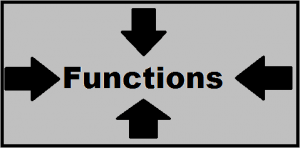[This article was first published on R-exercises, and kindly contributed to R-bloggers]. (You can report issue about the content on this page here)
Want to share your content on R-bloggers? click here if you have a blog, or here if you don't.Higher order functions are functions that take other functions as arguments or return functions as their result. In this set of exercises we will focus on the former. R has a set of built-in higher order functions: `Map`, `Reduce`, `Filter`, `Find`, `Position`, `Negate`. They enable us to complete complex operations by using simple single-purpose functions as their building blocks. In R this is especially helpful in cases where we cannot depend on vectorization and have to utilize control statements like for loops. In such scenarios higher order functions help us by: a) simplifying and shortening the syntax, b) getting rid of counter indices and c) getting rid of temporary storage values.

Exercises in this section will have to be solved by using one or more of the higher order functions mentioned above. It might be useful reading their help page before continuing.

Answers to the exercises are available here.

If you obtained a different (correct) answer than those listed on the solutions page, please feel free to post your answer as a comment on that page.

Exercise 1
You are working on 3 datasets all at once:
`multidata <- list(mtcars, USArrests, rock)`
`summary(multidata[])` will return the summary information for a single dataset.
Obtain summary information for every dataset in the list.

Exercise 2
`cumsum(1:100)` returns the cumulative sums of a vector of numbers from 1 to 100.
Do the same using `sum` and an appropriate higher order function.

Exercise 3
You have a vector of numbers from 1 to 10. You want to multiply all those numbers first by 2 and then by 4. Why the following line does not work and how to fix it?
`Map(`*`, 1:10, c(2,4))`

Exercise 4
Expression `sample(LETTERS, 5, replace=TRUE)` obtains 5 random letters.
Generate a list with 10 elements, where first element contains 1 random letter, second element 2 random letters and so on.

Note: use a fixed random seed: `set.seed(14)`

Exercise 5
Library `spatstat` has a function `is.prime()` that checks if a given number is a prime.
Find all prime numbers between 100 and 200.

Exercise 6
We have a vector containing all the words of the English language –
`words <- scan("http://www-01.sil.org/linguistics/wordlists/english/wordlist/wordsEn.txt", what="character")`
a. Using a function that checks if a given words contains any vowels:
`containsVowel <- function(x) grepl("a|o|e|i|u", x)`
find all words that do not contain any vowels.
b. Using a function `is.colour()` from the `spatstat` library find the index of the first word inside the words vector corresponding to a valid R color.

Exercise 7
a. Find the smallest number between 10000 and 20000 that is divisible by 1234.
b. Find the largest number between 10000 and 20000 that is divisible by 1234.

Exercise 8
Consider the `babynames` dataset from the `babynames` library.
Start with a list containing the used names for each year:
`library(babynames); namesData <- split(babynames\$name, babynames\$year)`
a. Obtain a set of names that were present in every year.
b. Obtain a set of names that are only present in year 2014

Exercise 9
Using the same `babynames` dataset and a function that checks if word has more than 3 letters: `moreThan3 <- function(x) nchar(x) > 3`
Inside each year list leave only the names that have 3 letters or less.

Exercise 10
Using the same `babynames` dataset:
a. Split each name to a list of letters.
b. Join each list of letters by inserting an underscore “_” after each letter.

Note: if you have a word `x <- "exercise"` you can split it with `x2 <- strsplit(x, "")`
and join using underscores with `paste(x2[], collapse="_")`